# Existence of solutions of a system of 3D axisymmetric inviscid stagnation flows

## Abstract

A system of two integral equations is presented to describe the system of 3D axisymmetric inviscid stagnation flows related to Navier-Stokes equations and existence of its solutions is studied. Utilizing it, we construct analytically the similarity solutions of the 3D system. A nonexistence result is obtained. Previous study was only supported by numerical results.

MSC:34B18.

## 1 Introduction

The following system of two differential equations arising in the boundary layer problems in fluid mechanics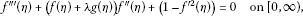(1.1)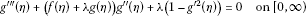(1.2)

with boundary conditions

$\begin{array}{r}f\left(0\right)=0,\phantom{\rule{2em}{0ex}}{f}^{\prime }\left(0\right)=0,\phantom{\rule{2em}{0ex}}{f}^{\prime }\left(\mathrm{\infty }\right)=1,\\ g\left(0\right)=0,\phantom{\rule{2em}{0ex}}{g}^{\prime }\left(0\right)=0,\phantom{\rule{2em}{0ex}}{g}^{\prime }\left(\mathrm{\infty }\right)=1\end{array}$
(1.3)

has been used to describe the system of 3D axisymmetric inviscid stagnation flow [1, 2], which consists of three partial differential equations [2, 3], where λ is a parameter related to the external flow components.

A solution of (1.1)-(1.3) is called a similarity solution and can be used to express the solutions of the 3D system. Regarding the study of (1.1)-(1.3), Howarth  presented a numerical study for the case $0<\lambda <1$ which can be applied to the stagnation region of an ellipsoid. Davey  investigated numerically the stagnation region near a saddle point ($-1<\lambda <0$). The two-dimensional cases, $\lambda =g=0$ or $\lambda =1$ and $g=f$, and the special cases of the Falkner-Skan equation were solved by Hiemenz  and by Homann , respectively. Regarding the Falkner-Skan problems, further analytical study can be found in . Also, one may refer to recent review of similarity solutions of the Navier-Stokes equations .

However, up to now, there has been very little analytical study on the existence of solutions of (1.1)-(1.3).

The main aim of this paper is to study the existence of solutions of (1.1)-(1.3) analytically for the case of $|\lambda |<1$. The method is to present a system of two integral equations and study the existence of its solutions and then use it to construct the solutions of (1.1)-(1.3). Also, a nonexistence result is obtained.

## 2 A system of two integral equations related to (1.1)-(1.3)

In this section, we present a system of two integral equations to describe a system of (1.1)-(1.3) under suitable conditions, which will be utilized in Section 4.

Let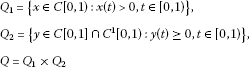and

$\mathrm{\Gamma }=\left\{\left(f,g\right)\in {C}^{3}\left[0,\mathrm{\infty }\right)×{C}^{3}\left[0,\mathrm{\infty }\right):{f}^{\prime }\left(\eta \right)\ge 0,{g}^{″}\left(\eta \right)>0,\eta \in \left[0,\mathrm{\infty }\right)\right\}.$

Lemma 2.1 If$\left(f,g\right)\in \mathrm{\Gamma }$is a solution of (1.1)-(1.3), then${g}^{″}\left(\mathrm{\infty }\right)=0$.

Proof Since ${g}^{\prime }\left(+\mathrm{\infty }\right)=1$, we have

$\underset{\eta \to \mathrm{\infty }}{lim inf}{g}^{″}\left(\eta \right)=0.$
(2.1)

Notice that $\left(f,g\right)\in \mathrm{\Gamma }$, $f\left(\eta \right)={\int }_{0}^{\eta }{f}^{\prime }\left(s\right)\phantom{\rule{0.2em}{0ex}}ds\ge 0$, ${g}^{\prime }\left(\eta \right)={\int }_{0}^{\eta }{g}^{″}\left(s\right)\phantom{\rule{0.2em}{0ex}}ds\ge 0$, $g\left(\eta \right)={\int }_{0}^{\eta }{g}^{\prime }\left(s\right)\phantom{\rule{0.2em}{0ex}}ds>0$ and $1>{g}^{\prime }\left(\eta \right)>0$ for $\eta \in \left(0,+\mathrm{\infty }\right)$.

If $\lambda \ge 0$, we know ${g}^{‴}\left(\eta \right)=-\left(f\left(\eta \right)+\lambda g\left(\eta \right)\right){g}^{″}\left(\eta \right)-\lambda \left(1-{g}^{\mathrm{\prime }2}\left(\eta \right)\right)\le 0$ and then ${g}^{″}$ is decreasing on $\left[0,+\mathrm{\infty }\right)$, which implies that ${lim}_{\eta \to \mathrm{\infty }}{g}^{″}\left(\eta \right)$ exists. Hence, ${g}^{″}\left(\mathrm{\infty }\right)=0$ by (2.1).

If $\lambda <0$, we have ${g}^{\mathrm{\prime }\mathrm{\prime }\mathrm{\prime }}\left(0\right)=-\lambda >0$ by (1.2). By (2.1), there exists ${\eta }_{0}>0$ such that ${g}^{″}\left({\eta }_{0}\right)<{g}^{″}\left(0\right)$ and then there exists ${\eta }^{\ast }$ such that ${g}^{″}\left({\eta }^{\ast }\right)=max\left\{{g}^{″}\left(\eta \right):\eta \in \left[0,{\eta }_{0}\right]\right\}$. Obviously, ${\eta }^{\ast }\in \left(0,{\eta }_{0}\right]$ by ${g}^{\mathrm{\prime }\mathrm{\prime }\mathrm{\prime }}\left(0\right)>0$. We prove that ${g}^{″}$ is decreasing on $\left({\eta }^{\ast },\mathrm{\infty }\right)$.

In fact, if there exist ${\eta }_{1},{\eta }_{2}\in \left({\eta }^{\ast },+\mathrm{\infty }\right)$ with ${\eta }_{1}<{\eta }_{2}$ such that ${g}^{″}\left({\eta }_{1}\right)<{g}^{″}\left({\eta }_{2}\right)$. Let ${\eta }_{\ast }\in \left[{\eta }^{\ast },{\eta }_{2}\right]$ such that ${g}^{″}\left({\eta }_{\ast }\right)=min\left\{{g}^{″}\left(\eta \right):\eta \in \left[{\eta }^{\ast },{\eta }_{2}\right]\right\}>0$, then ${g}^{‴}\left({\eta }_{\ast }\right)=0$ and ${g}^{\left(4\right)}\left({\eta }_{\ast }\right)\ge 0$.

Differentiating (1.2) with η, we have

${g}^{\left(4\right)}\left(\eta \right)=\left(\lambda {g}^{\prime }\left(\eta \right)-{f}^{\prime }\left(\eta \right)\right){g}^{″}\left(\eta \right)-\left(f\left(\eta \right)+\lambda g\left(\eta \right)\right){g}^{‴}\left(\eta \right),$

then

${g}^{\left(4\right)}\left({\eta }_{\ast }\right)=\left(\lambda {g}^{\prime }\left({\eta }_{\ast }\right)-{f}^{\prime }\left({\eta }_{\ast }\right)\right){g}^{″}\left({\eta }_{\ast }\right)<0,$

a contradiction. Hence, ${g}^{″}\left(\eta \right)$ is decreasing on $\left({\eta }^{\ast },+\mathrm{\infty }\right)$ and then ${g}^{″}\left(\mathrm{\infty }\right)=0$.

This completes the proof. □

Theorem 2.1 If$\left(f,g\right)\in \mathrm{\Gamma }$is a solution of (1.1)-(1.2), then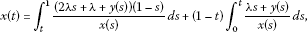(2.2)(2.3)

has a solution$\left(x,y\right)\in Q$, where${G}_{0,1}\left(t,s\right)$denotes the Green function for${u}^{″}\left(t\right)=0$with$u\left(0\right)=0$and$u\left(b\right)=0$defined by

${G}_{0,b}\left(t,s\right)=\left\{\begin{array}{cc}t\left(b-s\right)/b,\hfill & 0\le t\le s\le b,\hfill \\ s\left(b-t\right)/b,\hfill & 0\le s\le t\le b.\hfill \end{array}$
(2.4)

Proof Assume that $\left(f,g\right)\in \mathrm{\Gamma }$. Let $\eta :=\eta \left(t\right)={\left({g}^{\prime }\right)}^{-1}\left(t\right)$ for $t\in \left[0,1\right)$ be the inverse function to $t={g}^{\prime }\left(\eta \right):\left[0,\mathrm{\infty }\right)\to \left[0,1\right)$. It follows that ${g}^{\prime }$ is strictly increasing on $\left[0,+\mathrm{\infty }\right)$ and $\eta \left(t\right)={\left({g}^{\prime }\right)}^{-1}\left(t\right):\left[0,1\right)\to \left[0,\mathrm{\infty }\right)$ with ${\left({g}^{\prime }\right)}^{-1}\left(0\right)=0$, ${lim}_{t\to {1}^{-}}{\left({g}^{\prime }\right)}^{-1}\left(t\right)=\mathrm{\infty }$. Let $x\left(t\right)={g}^{″}\left(\eta \right)>0$ for $t\in \left[0,1\right)$, by Lemma 2.1, $x\left(1\right)={lim}_{\eta \to \mathrm{\infty }}{g}^{″}\left(\eta \right)=0$. This implies that $x\left(t\right)>0$ for $t\in \left[0,1\right)$ and x is continuous on $\left[0,1\right)$. By Lemma 2.1, we see that x is continuous from the left at 1. Hence, we have $x\left(t\right)\in C\left[0,1\right]$ and $x\left(1\right)=0$, i.e., $x\left(t\right)\in {Q}_{1}$.

Using the chain rule to $x\left(t\right)={g}^{″}\left(\eta \right)$, we obtain ${g}^{‴}\left(\eta \right)\frac{d\eta }{dt}={x}^{\prime }\left(t\right)$ and by the inverse function theorem, we have

This, together with ${g}^{\prime }\left(\eta \right)=t$, implies

Integrating the last equality from 0 to t implies

Let

Then $y\left(0\right)=0$. By ${f}^{\prime }\left(\mathrm{\infty }\right)=1$, we know that y is continuous from the left at 1 and then $y\left(1\right)=1$.

Notice that ${f}^{\prime }\left(\eta \right)\frac{d\eta }{dt}=\frac{y\left(t\right)}{x\left(t\right)}$, $t\in \left[0,1\right)$, we have $f\left(\eta \right)={\int }_{0}^{t}\frac{y\left(s\right)}{x\left(s\right)}\phantom{\rule{0.2em}{0ex}}ds$.

Differentiating $y\left(t\right)$ with t, we have

From this, we have ${f}^{″}\left(\eta \right)={y}^{\prime }\left(t\right)x\left(t\right)$ for $\eta \in \left[0,\mathrm{\infty }\right)$ and $y\in {Q}_{2}$.

Differentiating ${f}^{″}\left(\eta \right)$ with t and utilizing $\frac{d\eta }{dt}=\frac{1}{x\left(t\right)}$, we have

$\frac{{f}^{‴}\left(\eta \right)}{x\left(t\right)}={y}^{″}\left(t\right)x\left(t\right)+{y}^{\prime }\left(t\right){x}^{\prime }\left(t\right).$

Hence,

${f}^{‴}\left(\eta \right)={y}^{″}\left(t\right){x}^{2}\left(t\right)+{y}^{\prime }\left(t\right)x\left(t\right){x}^{\prime }\left(t\right).$

Substituting g, ${g}^{\prime }$, ${g}^{″}$, ${g}^{‴}$ and f into (1.2) implies

${x}^{\prime }\left(t\right)=-{\int }_{0}^{t}\frac{y\left(s\right)+\lambda s}{x\left(s\right)}\phantom{\rule{0.2em}{0ex}}ds+\frac{\lambda \left({t}^{2}-1\right)}{x\left(t\right)},\phantom{\rule{1em}{0ex}}t\in \left[0,1\right).$
(2.5)

Integrating (2.5) from t to 1, we have

$\begin{array}{rcl}x\left(1\right)-x\left(t\right)& =& -{\int }_{t}^{1}{\int }_{0}^{\sigma }\frac{y\left(s\right)+\lambda s}{x\left(s\right)}\phantom{\rule{0.2em}{0ex}}ds\phantom{\rule{0.2em}{0ex}}d\sigma +{\int }_{t}^{1}\frac{\lambda \left({s}^{2}-1\right)}{x\left(s\right)}\phantom{\rule{0.2em}{0ex}}ds\\ =& {\int }_{t}^{1}\frac{\lambda \left({s}^{2}-1\right)}{x\left(s\right)}\phantom{\rule{0.2em}{0ex}}ds-{\int }_{0}^{t}\left({\int }_{t}^{1}\frac{y\left(s\right)+\lambda s}{x\left(s\right)}\phantom{\rule{0.2em}{0ex}}d\sigma \right)\phantom{\rule{0.2em}{0ex}}ds-{\int }_{t}^{1}\left({\int }_{s}^{1}\frac{y\left(s\right)+\lambda s}{x\left(s\right)}\phantom{\rule{0.2em}{0ex}}d\sigma \right)\phantom{\rule{0.2em}{0ex}}ds\\ =& {\int }_{t}^{1}\frac{\lambda \left({s}^{2}-1\right)}{x\left(s\right)}\phantom{\rule{0.2em}{0ex}}ds-{\int }_{0}^{t}\frac{y\left(s\right)+\lambda s}{x\left(s\right)}\left(1-t\right)\phantom{\rule{0.2em}{0ex}}ds-{\int }_{t}^{1}\frac{\left(y\left(s\right)+\lambda s\right)\left(1-s\right)}{x\left(s\right)}\phantom{\rule{0.2em}{0ex}}ds\\ =& {\int }_{t}^{1}\frac{\lambda \left({s}^{2}-1\right)-\left(\lambda s+y\left(s\right)\right)\left(1-s\right)}{x\left(s\right)}\phantom{\rule{0.2em}{0ex}}ds-\left(1-t\right){\int }_{0}^{t}\frac{\lambda s+y\left(s\right)}{x\left(s\right)}\phantom{\rule{0.2em}{0ex}}ds\\ =& {\int }_{t}^{1}\frac{\left(2\lambda s+\lambda +y\left(s\right)\right)\left(s-1\right)}{x\left(s\right)}\phantom{\rule{0.2em}{0ex}}ds-\left(1-t\right){\int }_{0}^{t}\frac{\lambda s+y\left(s\right)}{x\left(s\right)}\phantom{\rule{0.2em}{0ex}}ds.\end{array}$

By $x\left(1\right)=0$, then

$x\left(t\right)={\int }_{t}^{1}\frac{\left(2\lambda s+\lambda +y\left(s\right)\right)\left(1-s\right)}{x\left(s\right)}\phantom{\rule{0.2em}{0ex}}ds+\left(1-t\right){\int }_{0}^{t}\frac{\lambda s+y\left(s\right)}{x\left(s\right)}\phantom{\rule{0.2em}{0ex}}ds.$

Substituting f, ${f}^{\prime }$, ${f}^{″}$, ${f}^{‴}$ and g into (1.1) implies

${y}^{″}\left(t\right){x}^{2}\left(t\right)+{y}^{\prime }\left(t\right)x\left(t\right){x}^{\prime }\left(t\right)+{y}^{\prime }\left(t\right)x\left(t\right){\int }_{0}^{t}\frac{\lambda s+y\left(s\right)}{x\left(s\right)}\phantom{\rule{0.2em}{0ex}}ds+\left(1-{y}^{2}\left(t\right)\right)=0.$

By ${\int }_{0}^{t}\frac{\lambda s+y\left(s\right)}{x\left(s\right)}\phantom{\rule{0.2em}{0ex}}ds=\frac{\lambda \left({t}^{2}-1\right)}{x\left(t\right)}-{x}^{\prime }\left(t\right)$, we have

${y}^{″}\left(t\right)+\frac{\lambda \left({t}^{2}-1\right){y}^{\prime }\left(t\right)+\left(1-{y}^{2}\left(t\right)\right)}{{x}^{2}\left(t\right)}=0.$

Therefore,

$y\left(t\right)={\int }_{0}^{1}{G}_{0,1}\left(t,s\right)\frac{\lambda \left({s}^{2}-1\right){y}^{\prime }\left(s\right)+\left(1-{y}^{2}\left(s\right)\right)}{{x}^{2}\left(s\right)}\phantom{\rule{0.2em}{0ex}}ds+t,\phantom{\rule{1em}{0ex}}t\in \left[0,1\right),$

where ${G}_{0,1}\left(t,s\right)$ is defined by (2.4). Hence, $\left(x,y\right)$ is a solution of (2.2)-(2.3) in Q. □

## 3 Positive solutions of the system (2.2)-(2.3)

In this section, we will use the fixed point theorem to study the existence of positive solutions of the system (2.2)-(2.3).

Let

$\delta =\delta \left(\lambda \right)=-\frac{\lambda }{2\lambda +1},\phantom{\rule{1em}{0ex}}\lambda \in \left(-\frac{1}{3},0\right].$

It is easy to verify

$0<\delta <1\phantom{\rule{1em}{0ex}}\text{if and only if}\phantom{\rule{1em}{0ex}}-\frac{1}{3}<\lambda <0.$

We define some functions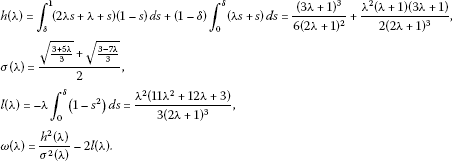By computation, $\omega \left(0\right)=\frac{1}{36}$, $\omega \left(-\frac{1}{3}\right)=-\frac{4}{9}$, there exists ${\lambda }_{0}\in \left(-\frac{1}{3},0\right)$ such that $\omega \left(\lambda \right)>0$ for $\lambda \in \left({\lambda }_{0},0\right]$ and $\omega \left({\lambda }_{0}\right)=0$.

In order to study the existence of solutions of (2.2)-(2.3) in Q for $\lambda \in \left({\lambda }_{0},1\right)$, we denote the norm of the Banach space $C\left[0,1\right]×{C}^{1}\left[0,1\right]$ by

$\parallel \left(x,y\right)\parallel =\parallel x\parallel +\parallel y\parallel +\parallel {y}^{\prime }\parallel ,$

where $\parallel x\parallel =max\left\{|x\left(t\right)|:t\in \left[0,1\right]\right\}$.

Let $\left(x,y\right)\in C\left[0,1\right]×{C}^{1}\left[0,1\right]$ and $n>0$ be a natural number, we define

$\phi x\left(t\right)=max\left\{x\left(t\right),c\left(t\right)\right\},\phantom{\rule{2em}{0ex}}{\phi }_{n}x\left(t\right)=max\left\{x\left(t\right),c\left(t\right),\frac{1}{n}\right\},\phantom{\rule{2em}{0ex}}\theta y\left(t\right)=max\left\{y\left(t\right),t\right\},$

where $c\left(t\right)={c}_{\lambda }\left(1-t\right)$, $t\in \left[0,1\right]$,

${c}_{\lambda }=\left\{\begin{array}{cc}\frac{1}{n},\hfill & \lambda \ge 0,\hfill \\ min\left\{\sqrt{h\left(\lambda \right)},\sqrt{\omega \left(\lambda \right)},\frac{\left(1+\lambda \right)\left(1-\delta \right){\delta }^{2}}{4\sigma \left(\lambda \right)}\right\},\hfill & {\lambda }_{0}<\lambda <0.\hfill \end{array}$
(3.1)

Notation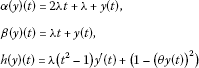and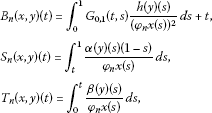where ${G}_{0,1}\left(t,s\right)$ is defined by (2.4).

Let $\left(x,y\right)\in C\left[0,1\right]×{C}^{1}\left[0,1\right]$, we define an operator F as follows:

${F}_{n}\left(x,y\right)\left(t\right)=\left({A}_{n}\left(x,y\right)\left(t\right),{B}_{n}\left(x,y\right)\left(t\right)\right),$

where

${A}_{n}\left(x,y\right)\left(t\right)={S}_{n}\left(x,y\right)\left(t\right)+\left(1-t\right){T}_{n}\left(x,y\right)+\frac{1}{n}.$

It is easy to verify that ${\phi }_{n}$, θ are continuous operators from $C\left[0,1\right]$ into $C\left[0,1\right]$ and ${\phi }_{n}x\left(t\right)\ge \frac{1}{n}$, $t\in \left[0,1\right]$, we know the following proposition holds:

Lemma 3.1${F}_{n}$is a continuous and compact operator from$C\left[0,1\right]×{C}^{1}\left[0,1\right]$to$C\left[0,1\right]×{C}^{1}\left[0,1\right]$.

Lemma 3.2 Let $\left(\lambda ,z,w\right)\in \left(-1,1\right)×C\left[0,1\right]×{C}^{1}\left[0,1\right]$ and $0<\mu \le 1$ such that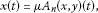(3.2)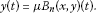(3.3)

Then the following assertions hold:

1. (i)

$\mu t\le y\left(t\right)\le 1$ for $t\in \left[0,1\right]$.

2. (ii)

${\int }_{0}^{1}|{y}^{\prime }\left(s\right)|\phantom{\rule{0.2em}{0ex}}ds\le 2$ and ${V}_{0}^{1}\left(y\right)\le 2$, where ${V}_{0}^{1}\left(y\right)$ is a total variation of y on $\left[0,1\right]$.

3. (iii)

If $\mu =1$, then $y\left(t\right)$ is increasing on $\left(0,1\right)$ and then $\theta y\left(t\right)=y\left(t\right)$ for $t\in \left[0,1\right]$.

Proof We shall use the basic fact: let $u\left(t\right)\in C\left[a,b\right]×{C}^{2}\left(a,b\right)$ and $u\left(\xi \right)$ ($\xi \in \left(a,b\right)$) be local minimum (maximum), then ${u}^{″}\left(\xi \right)\ge 0$ (≤0).

1. (i)

If there exists ${t}_{0}\in \left(0,1\right)$ such that $y\left({t}_{0}\right)>1$, by $y\left(0\right)=0<\mu =y\left(1\right)$, we know that there exists ${t}_{\ast }\in \left(0,1\right)$ such that $y\left({t}_{\ast }\right)=max\left\{y\left(t\right):t\in \left[0,1\right]\right\}>1$. Differentiating (3.3) with t twice, we have

${y}^{″}\left(t\right)=-\mu \frac{h\left(y\right)\left(t\right)}{{\left({\phi }_{n}x\left(t\right)\right)}^{2}}.$
(3.4)

By ${y}^{\prime }\left({t}_{\ast }\right)=0$ and (3.4), we have

${y}^{″}\left({t}_{\ast }\right)=-\frac{\mu \left(1-{y}^{2}\left({t}_{\ast }\right)\right)}{{\left({\phi }_{n}x\left({t}_{\ast }\right)\right)}^{2}}>0,$

a contradiction. Hence, $y\left(t\right)\le 1$ for $t\in \left(0,1\right)$.

If there exists ${t}_{0}\in \left[0,1\right]$ such that $\mu {t}_{0}>y\left({t}_{0}\right)$, let $\tau \left(t\right)=\mu t-y\left(t\right)$, by $\tau \left(0\right)=0=\tau \left(1\right)$ and $\tau \left({t}_{0}\right)>0$, we may assume ${t}_{\ast }\in \left(0,1\right)$ such that $\tau \left({t}_{\ast }\right)=max\left\{\tau \left(t\right):t\in \left[0,1\right]\right\}$. This implies ${\tau }^{\prime }\left({t}_{\ast }\right)=0$, i.e., ${y}^{\prime }\left({t}_{\ast }\right)=\mu$, and ${\tau }^{″}\left({t}_{\ast }\right)\le 0$. By (3.4) and $\theta y\left({t}_{\ast }\right)={t}_{\ast }$, we know

$h\left(y\right)\left({t}_{\ast }\right)=\lambda \left({t}_{\ast }^{2}-1\right)\mu +\left(1-{t}_{\ast }^{2}\right)=\left(1-\lambda \mu \right)\left(1-{t}_{\ast }^{2}\right)>0,$

then

${\tau }^{″}\left({t}_{\ast }\right)=-{y}^{″}\left({t}_{\ast }\right)=\frac{\mu h\left(y\right)\left({t}_{\ast }\right)}{{\left({\phi }_{n}x\left({t}_{\ast }\right)\right)}^{2}}>0,$

1. (ii)

Let $\stackrel{˜}{t}\in \left[0,1\right]$ such that $y\left(\stackrel{˜}{t}\right)=max\left\{y\left(t\right):t\in \left[0,1\right]\right\}$ and $\gamma =sup\left\{\stackrel{˜}{t}\right\}$. If $\gamma <1$, we prove that $y\left(t\right)$ is increasing on $\left(0,\gamma \right)$ and decreasing on $\left(\gamma ,1\right)$.

Since $y\left(0\right)=0$ and $y\left(1\right)=\mu >0$, then $\gamma >0$. Let $\gamma <1$. If there exist ${t}_{1},{t}_{2}\in \left(0,\gamma \right)$ with ${t}_{1}<{t}_{2}$ such that $y\left({t}_{1}\right)>y\left({t}_{2}\right)$, let ${t}_{\ast }\in \left({t}_{1},\gamma \right)$ such that $y\left({t}_{\ast }\right)=min\left\{y\left(t\right):t\in \left[{t}_{1},\gamma \right]\right\}$, then $y\left({t}_{\ast }\right)<1$ by (i). From ${y}^{\prime }\left({t}_{\ast }\right)=0$, ${t}_{\ast }\le \theta y\left({t}_{\ast }\right)<1$ and (3.4), we know

${y}^{″}\left({t}_{\ast }\right)=-\frac{\mu \left(1-{\left(\theta y\left({t}_{\ast }\right)\right)}^{2}\right)}{{\left({\phi }_{n}x\left({t}_{\ast }\right)\right)}^{2}}<0,$

If there exist ${t}_{1},{t}_{2}\in \left(\gamma ,1\right)$ with ${t}_{1}<{t}_{2}$ such that $y\left({t}_{1}\right), let ${t}_{\ast }\in \left(\gamma ,{t}_{2}\right)$ such that $y\left({t}_{\ast }\right)=min\left\{y\left(t\right):t\in \left[\gamma ,{t}_{2}\right]\right\}$, then $y\left({t}_{\ast }\right)<1$ by (i). Analogously, we know easily

${y}^{″}\left({t}_{\ast }\right)=-\frac{\mu \left(1-{\left(\theta y\left({t}_{\ast }\right)\right)}^{2}\right)}{{\left({\phi }_{n}x\left({t}_{\ast }\right)\right)}^{2}}<0,$

${\int }_{0}^{1}|{y}^{\prime }\left(s\right)|\phantom{\rule{0.2em}{0ex}}ds={\int }_{0}^{\gamma }{y}^{\prime }\left(s\right)\phantom{\rule{0.2em}{0ex}}ds-{\int }_{\gamma }^{1}{y}^{\prime }\left(s\right)\phantom{\rule{0.2em}{0ex}}ds=2y\left(\gamma \right)-\mu \le 2,$

and ${V}_{0}^{1}\left(y\right)={\int }_{0}^{1}|{y}^{\prime }\left(s\right)|\phantom{\rule{0.2em}{0ex}}ds\le 2$, i.e., (ii) holds.

1. (iii)

Let $\mu =1$. By (i) and $y\left(1\right)=1$, we know $\gamma =1$ and then $y\left(t\right)$ is increasing on $\left(0,1\right)$ and then $\theta y\left(t\right)=y\left(t\right)$ for $t\in \left[0,1\right]$. Hence, (iii) holds. □

Lemma 3.3

Let E be a Banach space, D be a bounded open set of E and$\theta \in D$, $F:\overline{D}\to E$is compact. If$x\ne \mu Fx$for any$0<\mu <1$and$x\in \partial D$, then F has a fixed point in$\overline{D}$.

Lemma 3.4 Let$\lambda \in \left(-1,1\right)$, then F has a fixed point$\left({x}_{n},{y}_{n}\right)$in$C\left[0,1\right]×{C}^{1}\left[0,1\right]$, i.e., there exists$\left({x}_{n},{y}_{n}\right)\in C\left[0,1\right]×{C}^{1}\left[0,1\right]$such that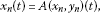(3.5)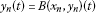(3.6)

hold.

Proof Let

$\mathrm{\Omega }=\left\{\left(x,y\right):\left(x,y\right)\in C\left[0,1\right]×{C}^{1}\left[0,1\right],\parallel \left(x,y\right)\parallel

where $R=16{n}^{2}$. We prove $\left(x,y\right)\ne \mu F\left(x,y\right)$ for $0<\mu <1$ and with $\parallel \left(x,y\right)\parallel =R$.

In fact, if there exist $\left(x,y\right)$ and μ with $\parallel \left(x,y\right)\parallel =R$ and $0<\mu <1$ such that $\left(x,y\right)\ne \mu F\left(x,y\right)$, by Lemma 3.2(i) and (iii), we have $\parallel y\parallel \le 1$.

Since $|\alpha \left(y\right)\left(s\right)|\le \left(2|\lambda |+|\lambda |+1\right)=3|\lambda |+1$ and $|\beta \left(y\right)\left(s\right)|\le |\lambda |+1$ for $s\in \left[0,1\right]$, this, together with $1-t\le 1-s$ for $s\le t$ and ${\phi }_{n}x\left(t\right)\ge \frac{1}{n}$, implies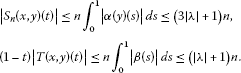And then $|x\left(t\right)|\le |{S}_{n}\left(x,y\right)\left(t\right)|+\left(1-t\right)|{T}_{n}\left(x,y\right)\left(t\right)|+1\le 2\left(2|\lambda |+1\right)n+1$, i.e., $\parallel x\parallel \le 2\left(2|\lambda |+1\right)n+1$.

By (3.3), we have

${y}^{\prime }\left(t\right)=-{\int }_{0}^{t}s\frac{h\left(y\right)\left(s\right)}{{\left({\phi }_{n}x\left(s\right)\right)}^{2}}\phantom{\rule{0.2em}{0ex}}ds+{\int }_{t}^{1}\left(1-s\right)\frac{h\left(y\right)\left(s\right)}{{\left({\phi }_{n}x\left(s\right)\right)}^{2}}\phantom{\rule{0.2em}{0ex}}ds+1.$
(3.7)

Noticing that $|h\left(y\right)\left(s\right)|\le |\lambda ||{y}^{\prime }\left(s\right)|+1$ and ${\phi }_{n}x\left(s\right)\ge \frac{1}{n}$ for $s\in \left[0,1\right]$, we obtain $|\frac{h\left(y\right)\left(s\right)}{{\left({\phi }_{n}x\left(s\right)\right)}^{2}}|\le {n}^{2}\left(|\lambda ||{y}^{\prime }\left(s\right)|+1\right)$ for $s\in \left[0,1\right]$. This, together with (3.7) and Lemma 3.2(ii), implies

$\begin{array}{rcl}|{y}^{\prime }\left(t\right)|& \le & {\int }_{0}^{1}|\frac{h\left(y\right)\left(s\right)}{{\left({\phi }_{n}x\left(s\right)\right)}^{2}}|\phantom{\rule{0.2em}{0ex}}ds+{\int }_{0}^{1}|\frac{h\left(y\right)\left(s\right)}{{\left({\phi }_{n}x\left(s\right)\right)}^{2}}|\phantom{\rule{0.2em}{0ex}}ds+1\\ \le & 2\left(2|\lambda |+1\right){n}^{2}+1,\end{array}$

i.e., $\parallel {y}^{\prime }\parallel \le 2\left(2|\lambda |+1\right){n}^{2}+1$. Hence,

$\begin{array}{rcl}\parallel \left(x,y\right)\parallel & =& \parallel x\parallel +\parallel y\parallel +\parallel {y}^{\prime }\parallel \\ \le & 2\left(2|\lambda |+1\right)n+1+2\left(2|\lambda |+1\right){n}^{2}+1

By Lemmas 3.1 and 3.3, F has a fixed point $\left({x}_{n},{y}_{n}\right)$ in $C\left[0,1\right]×{C}^{1}\left[0,1\right]$. □

Lemma 3.5 Let$\left({x}_{n},{y}_{n}\right)$be in Lemma  3.4, then

1. (i)

$\left\{{x}_{n}\left(t\right)\right\}$ is bounded on $\left[0,1\right]$.

2. (ii)

$\left\{{x}_{n}^{\prime }\left(t\right)\right\}$ is bounded on $\left[0,b\right]$ for any $b\in \left(\frac{1}{2},1\right)$.

Proof By Lemma 3.3(i), we know $0\le {y}_{n}\left(t\right)\le 1$. By (3.5), we have

${x}_{n}^{\prime }\left(t\right)=\frac{-\lambda \left(1-{t}^{2}\right)}{\phi {x}_{n}\left(t\right)}-{\int }_{0}^{t}\frac{{y}_{n}\left(s\right)+\lambda s}{\phi {x}_{n}\left(s\right)}\phantom{\rule{0.2em}{0ex}}ds,\phantom{\rule{1em}{0ex}}t\in \left[0,1\right).$
(3.8)
1. (i)

For $\lambda \ge 0$, we know ${x}_{n}^{\prime }\left(t\right)<0$ for $t\in \left[0,1\right]$, i.e., ${x}_{n}\left(t\right)$ is decreasing in $\left[0,1\right]$, by ${x}_{n}\left(1\right)=\frac{1}{n}$, $\phi {x}_{n}\left(t\right)={x}_{n}\left(t\right)$ for $t\in \left[0,1\right]$. By $\alpha \left({y}_{n}\right)\left(t\right)\ge t$ for $t\in \left[0,1\right]$ and (3.5), we have

${x}_{n}\left(t\right)\ge {S}_{n}\left({x}_{n},{y}_{n}\right)\left(t\right)\ge {\int }_{t}^{1}\frac{s}{{\phi }_{n}x\left(s\right)}\phantom{\rule{0.2em}{0ex}}ds\ge \frac{t}{{x}_{n}\left(t\right)}{\int }_{t}^{1}\left(1-s\right)\phantom{\rule{0.2em}{0ex}}ds.$
(3.9)

And then ${x}_{n}\left(t\right)\ge \frac{\left(1-t\right)\sqrt{2t}}{2}$ for $t\in \left[0,1\right]$. Obviously, ${x}_{n}\left(t\right)\ge \frac{1-t}{2}$ for $t\in \left[\frac{1}{2},1\right]$. This, together with the decrease in ${x}_{n}$, implies

(3.10)

Let ${c}^{\ast }\left(t\right)=\mu \left(1-t\right)$, μ defined by

where ${c}_{\lambda }$ defined in (3.1).

It is easy to verify ${\phi }_{n}{x}_{n}\left(t\right)\ge {c}^{\ast }\left(t\right)$ for $t\in \left[0,1\right]$. And then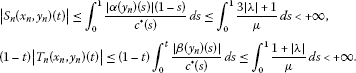The last two inequalities imply that $\left\{{x}_{n}\left(t\right)\right\}$ is bounded on $\left[0,1\right]$.

1. (ii)

By (3.8),

$|{x}_{n}^{\prime }\left(t\right)|\le \frac{|\lambda |\left(1+t\right)}{\mu }+{\int }_{0}^{t}\frac{2}{{c}^{\ast }\left(s\right)}\phantom{\rule{0.2em}{0ex}}ds,\phantom{\rule{1em}{0ex}}t\in \left[0,1\right),$

we know that $\left\{{x}_{n}^{\prime }\left(t\right)\right\}$ is bounded on $\left[0,b\right]$ for any $b\in \left(\frac{1}{2},1\right)$. □

Lemma 3.6 Let$\left({x}_{n},{y}_{n}\right)$be in Lemma  3.4, then

1. (i)

$t\le {y}_{n}\left(t\right)\le 1$ and ${y}_{n}\left(t\right)$ is increasing in $\left[0,1\right]$.

2. (ii)

$\left\{{y}_{n}^{\prime }\left(t\right)\right\}$ is bounded and equicontinuous in $\left[0,b\right]$ for any $b\in \left(\frac{1}{2},1\right)$.

Proof

1. (i)

Lemma 3.2(i) and (iii) imply the desired results.

2. (ii)

For $b\in \left(\frac{1}{2},1\right)$, let ${t}_{b}\in \left[0,b\right]$ such that ${y}_{n}^{\prime }\left({t}_{b}\right)=min\left\{{y}_{n}^{\prime }\left(t\right):t\in \left[0,b\right]\right\}$. Since ${y}_{n}^{\prime }\left(t\right)\ge 0$ on $\left[0,1\right]$, by Lemma 3.2(ii), ${y}_{n}^{\prime }\left({t}_{b}\right)b\le {\int }_{0}^{b}{y}_{n}^{\prime }\left(s\right)\phantom{\rule{0.2em}{0ex}}ds\le {\int }_{0}^{1}{y}_{n}^{\prime }\left(s\right)\phantom{\rule{0.2em}{0ex}}ds\le 2$, we obtain ${y}_{n}^{\prime }\left({t}_{b}\right)\le \frac{2}{b}$.

Differentiating (3.6) with t twice, we have ${y}_{n}^{″}\left(t\right)=-\frac{h\left({y}_{n}\right)\left(t\right)}{{\left({\phi }_{n}{x}_{n}\left(t\right)\right)}^{2}}$. Integrating this equality from 0 to $t\le b$, we have

${y}_{n}^{\prime }\left(t\right)-{y}_{n}^{\prime }\left({t}_{b}\right)=-{\int }_{{t}_{b}}^{t}\frac{h\left({y}_{n}\right)\left(s\right)}{{\left({\phi }_{n}{x}_{n}\left(s\right)\right)}^{2}}\phantom{\rule{0.2em}{0ex}}ds.$

Noticing that $|h\left({y}_{n}\right)\left(t\right)|\le |\lambda |{y}_{n}^{\prime }\left(t\right)+1$ and ${c}^{\ast }\left(t\right)\ge {c}^{\ast }\left(b\right)$ for $t\in \left[0,b\right]$ and Lemma 3.2(ii), we know

$|{y}_{n}^{\prime }\left(t\right)|\le \frac{2|\lambda |+1}{{\left({c}^{\ast }\left(b\right)\right)}^{2}}+|{y}_{n}^{\prime }\left({t}_{b}\right)|\le \frac{2|\lambda |+1}{{\left({c}^{\ast }\left(b\right)\right)}^{2}}+\frac{2}{b},$

i.e., $\left\{{y}_{n}^{\prime }\left(t\right)\right\}$ is bounded on $\left[0,b\right]$. Let ${M}_{b}=sup\left\{{M}_{n}\right\}$ (where ${M}_{n}=max\left\{{y}_{n}^{\prime }\left(t\right):t\in \left[0,b\right]\right\}$), we know

This implies that $\left\{{y}_{n}^{\prime }\left(t\right)\right\}$ is equicontinuous on $\left[0,b\right]$. □

Theorem 3.1 There exists $\left(x,y\right)\in C\left[0,1\right]×\left(C\left[0,1\right]\cap {C}^{1}\left[0,1\right)\right)$ such that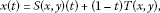(3.11)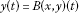(3.12)

hold, where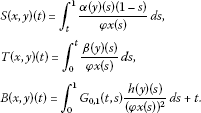Proof Let $\left({x}_{n},{y}_{n}\right)$ be in Lemma 3.4, by Lemma 3.5(ii) and (iii), we know that $\left\{{x}_{n}\left(t\right)\right\}$ is bounded and equicontinuous on $\left[0,b\right]$ for any $b\in \left(\frac{1}{2},1\right)$. Letting $b=1-\frac{1}{k}$ ($k=3,4,\dots$), utilizing the diagonal principle and the Arzela-Ascoli theorem, we know that there exists a subsequence $\left\{{x}_{{n}_{k}}\left(t\right)\right\}$ of $\left\{{x}_{n}\left(t\right)\right\}$ and $x\left(t\right)\in C\left[0,1\right)$ such that ${x}_{{n}_{k}}\left(t\right)$ converges to $x\left(t\right)$ for $t\in \left[0,1\right)$. Without loss of generality, we assume that $\left\{{x}_{{n}_{k}}\left(t\right)\right\}$ is itself of $\left\{{x}_{n}\left(t\right)\right\}$.

By Lemma 3.6, we know that $\left\{{y}_{n}^{\prime }\left(t\right)\right\}$ is bounded and equicontinuous on $\left[0,b\right]$ for any $b\in \left(\frac{1}{2},1\right)$ and then $\left\{{y}_{n}\left(t\right)\right\}$ is bounded and equicontinuous on $\left[0,b\right]$. Let $b=1-\frac{1}{k}$ ($k=3,4,\dots$), the diagonal principle and the Arzela-Ascoli theorem imply that there exist y and ${y}_{0}$ in $C\left[0,1\right)$ and two subsequences $\left\{{y}_{{n}_{k}}\left(t\right)\right\}$ and $\left\{{y}_{{n}_{i}}^{\prime }\left(t\right)\right\}$ with $\left\{{y}_{{n}_{i}}\left(t\right)\right\}\subseteq \left\{{y}_{{n}_{k}}\left(t\right)\right\}\subseteq \left\{{y}_{n}\left(t\right)\right\}$ such that ${y}_{{n}_{k}}\left(t\right)$ converges to $y\left(t\right)$ for $t\in \left[0,1\right)$ with $y\left(1\right)=1$ and ${y}_{{n}_{i}}^{\prime }\left(t\right)$ converges to ${y}_{0}\left(t\right)$ for each $t\in \left[0,1\right)$. For the sake of convenience, we assume that $\left\{{y}_{{n}_{i}}\left(t\right)\right\}$ and $\left\{{y}_{{n}_{k}}\left(t\right)\right\}$ are itself of $\left\{{y}_{n}\left(t\right)\right\}$. By ${y}_{n}\left(t\right)={\int }_{0}^{t}{y}_{n}^{\prime }\left(s\right)\phantom{\rule{0.2em}{0ex}}ds$, we obtain $y\left(t\right)={\int }_{0}^{t}{y}_{0}\left(s\right)\phantom{\rule{0.2em}{0ex}}ds$ and then ${y}_{0}\left(t\right)={y}^{\prime }\left(t\right)$ for $t\in \left[0,1\right)$.

Since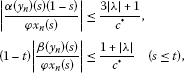$\alpha \left({y}_{n}\right)\left(t\right)$ converges to $\alpha \left(y\right)\left(t\right)$ and $\beta \left({y}_{n}\right)\left(t\right)$ converges to $\beta \left(y\right)\left(t\right)$ for $t\in \left[0,1\right)$, by the Lebesgue dominated theorem (the dominated function $F\left(s\right)=\frac{3|\lambda |+1}{{c}^{\ast }}$, $s\in \left[0,1\right]\right)$, we have that $\left(x,y\right)$ satisfies (3.11) and $x\in {Q}_{1}$.

Fix $t\in \left(0,1\right)$ and choose $b\in \left(0,1\right)$ such that $t\le b$, then

Noticing that $|h\left({y}_{n}\right)\left(s\right)|\le |\lambda ||{y}_{n}^{\prime }\left(s\right)|+1\le |\lambda |{M}_{b}+1$ and $h\left({y}_{n}\right)\left(s\right)$ converges to $h\left(y\right)\left(s\right)$ for $s\in \left[0,b\right]$, by the Lebesgue dominated theorem (the dominated function $F\left(s\right)=\frac{{M}_{b}+1}{{\left({c}^{\ast }\left(b\right)\right)}^{2}}$ on $\in \left[0,b\right]$), we have

Differentiating the last equality twice, we know

By (i), we know $t\le y\left(t\right)\le 1$ and ${lim}_{t\to 1}y\left(t\right)=1=y\left(1\right)$ and then $y\in C\left[0,1\right]\cap {C}^{1}\left[0,1\right)$. This, together with (2.4), implies that $y\left(t\right)$ satisfies (3.12). Clearly, $\left(x,y\right)\in Q$. □

Theorem 3.2 For$\lambda \in \left({\lambda }_{0},1\right)$, the system (2.2)-(2.3) has at least a solution$\left(x,y\right)$in Q.

Proof Let $\left(x,y\right)$ in Theorem 3.1. It is clear that we only prove $\phi x\left(t\right)=x\left(t\right)$. If $\lambda \ge 0$, by (3.10), we obtain $x\left(t\right)\ge \frac{1-t}{4}$ for $t\in \left[0,1\right]$ and then $\phi x\left(t\right)=x\left(t\right)$. Next, we prove $x\left(t\right)\ge {c}_{\lambda }\left(1-t\right)$ for $t\in \left[0,1\right]$ for ${\lambda }_{0}<\lambda <0$.

Let $\gamma \in \left[0,1\right]$ such that $M=\phi x\left(\gamma \right)=max\left\{\phi x\left(t\right):t\in \left[0,1\right]\right\}$, then

$\begin{array}{rcl}M& \ge & x\left(\delta \right)={\int }_{\delta }^{1}\frac{\left(2\lambda s+\lambda +y\left(s\right)\right)\left(1-s\right)}{\phi x\left(s\right)}+\left(1-\delta \right){\int }_{0}^{\delta }\frac{\lambda s+y\left(s\right)}{\phi x\left(s\right)}\phantom{\rule{0.2em}{0ex}}ds\\ \ge & {\int }_{\delta }^{1}\frac{\left(2\lambda s+\lambda +s\right)\left(1-s\right)}{max\left\{M,{c}_{\lambda }\right\}}\phantom{\rule{0.2em}{0ex}}ds+\left(1-\delta \right){\int }_{0}^{\delta }\frac{\lambda s+s}{max\left\{M,{c}_{\lambda }\right\}}\phantom{\rule{0.2em}{0ex}}ds\\ =& \frac{1}{max\left\{M,{c}_{\lambda }\right\}}{\int }_{\delta }^{1}\left(2\lambda s+\lambda +s\right)\left(1-s\right)\phantom{\rule{0.2em}{0ex}}ds+\left(1-\delta \right){\int }_{0}^{\delta }\left(\lambda s+s\right)\phantom{\rule{0.2em}{0ex}}ds\\ =& \frac{h\left(\lambda \right)}{max\left\{M,{c}_{\lambda }\right\}}.\end{array}$

From this and ${c}_{\lambda }\le \sqrt{h\left(\lambda \right)}$, we obtain $M\ge \sqrt{h\left(\lambda \right)}$ and $x\left(\gamma \right)=\phi x\left(\gamma \right)=M$.

Let $S\left(t\right)=S\left(x,y\right)\left(t\right)$ and $S=max\left\{S\left(t\right):t\in \left[0,1\right]\right\}$, we prove

$S\le \sqrt{\frac{3+5\lambda }{3}}.$
(3.13)

By $\alpha \left(y\right)\left(0\right)=\lambda <0$ and $\alpha \left(y\right)\left(1\right)=3\lambda +1>0$, there exists ${t}_{0}\in \left(0,1\right)$ such that $\alpha \left(y\right)\left({t}_{0}\right)=0$. Since $\alpha {\left(y\right)}^{″}\left(t\right)={y}^{″}\left(t\right)\le 0$ for $t\in \left[0,1\right]$, i.e., $\alpha \left(y\right)\left(t\right)$ is concave down on $\left[0,1\right]$, then $\alpha \left(y\right)\left(s\right)\le 0$ for $s\in \left[0,{t}_{0}\right]$ and $\alpha \left(y\right)\left(s\right)\ge 0$ for $s\in \left[{t}_{0},1\right]$. Hence, $S=S\left({t}_{0}\right)$.

By (3.11), we have

we know

Integrating the last inequality from ${t}_{0}$ to 1 and utilizing $S\left(1\right)=0$, we have

$\frac{{S}^{2}\left({t}_{0}\right)}{2}\le {\int }_{{t}_{0}}^{1}\left(2\lambda s+\lambda +1\right)\left(1-s\right)\phantom{\rule{0.2em}{0ex}}ds\le {\int }_{0}^{1}\left(2\lambda s+\lambda +1\right)\left(1-s\right)\phantom{\rule{0.2em}{0ex}}ds=\frac{3+5\lambda }{6}.$

Hence, (3.13) holds.

By ${x}^{\prime }\left(0\right)>0$, $x\left(\delta \right)>0$ and $x\left(1\right)=0$, we have $0<\gamma <1$ and ${x}^{\prime }\left(\gamma \right)=0$, then

$0={x}^{\prime }\left(\gamma \right)=-\frac{\lambda \left(1-{\gamma }^{2}\right)}{\phi x\left(\gamma \right)}-{\int }_{0}^{\gamma }\frac{\lambda s+y\left(s\right)}{\phi x\left(s\right)}\phantom{\rule{0.2em}{0ex}}ds,$

i.e.,

${\int }_{0}^{\gamma }\frac{\lambda s+y\left(s\right)}{\phi x\left(s\right)}\phantom{\rule{0.2em}{0ex}}ds=-\frac{\lambda \left(1-{\gamma }^{2}\right)}{\phi x\left(\gamma \right)}.$

Hence,

$\left(1-\gamma \right)T\left(x,y\right)\left(\gamma \right)=-\frac{\lambda \left(1-\gamma \right)\left(1-{\gamma }^{2}\right)}{\phi x\left(\gamma \right)}\le -\frac{\lambda }{M}.$

This, together with (3.13), implies

$M=x\left(\gamma \right)=S\left(x,y\right)\left(\gamma \right)+\left(1-\gamma \right)T\left(x,y\right)\left(\gamma \right)\le \sqrt{\frac{3+5\lambda }{3}}-\frac{\lambda }{M},$

i.e.,

$M\le \frac{\sqrt{\frac{3+5\lambda }{3}}+\sqrt{\frac{3-7\lambda }{3}}}{2}=\sigma \left(\lambda \right).$

Since $\alpha \left(y\right)\left(t\right)\ge 2\lambda t+\lambda +t\ge 0$ for $t\in \left[\delta ,1\right]$, we have

$\begin{array}{rcl}x\left(t\right)& \ge & \left(1-t\right)T\left(x,y\right)\left(t\right)\ge \left(1-t\right)T\left(x,y\right)\left(\delta \right)\\ \ge & \left(1-t\right){\int }_{0}^{\delta }\frac{\lambda s+s}{\sigma \left(\lambda \right)}\phantom{\rule{0.2em}{0ex}}ds\ge \frac{\left(\lambda +1\right){\delta }^{2}}{2\sigma \left(\lambda \right)}\left(1-t\right),\phantom{\rule{1em}{0ex}}t\in \left[\delta ,1\right].\end{array}$

And then $x\left(t\right)\ge {c}_{\lambda }\left(t\right)$ for $t\in \left[\delta ,1\right]$.

Finally, we prove $x\left(t\right)\ge {c}_{\lambda }$ for $t\in \left[0,\delta \right]$.

In fact, if there exists $t\in \left[0,\delta \right]$ such that $x\left(t\right)<{c}_{\lambda }$, by $x\left(\delta \right)>{c}_{\lambda }$, there exists ${t}^{\prime }\in \left(0,\delta \right)$ such that $x\left(t\right)>{c}_{\lambda }$ for $t\in \left({t}^{\prime },\delta \right]$ and $x\left({t}^{\prime }\right)={c}_{\lambda }$.

From

$x\left(\delta \right)=S\left(x,y\right)\left(\delta \right)+\left(1-\delta \right)T\left(x,y\right)\left(\delta \right),$

$S\left(x,y\right)\left(\delta \right)\ge {\int }_{\delta }^{1}\frac{2\lambda s+\lambda +s}{\sigma \left(\lambda \right)}\phantom{\rule{0.2em}{0ex}}ds$ and $T\left(x,y\right)\left(\delta \right)\ge {\int }_{0}^{\delta }\frac{\lambda s+s}{\sigma \left(\lambda \right)}\phantom{\rule{0.2em}{0ex}}ds$, we obtain

$x\left(\delta \right)\ge \frac{h\left(\lambda \right)}{\sigma \left(\lambda \right)}.$

By (3.11), we have

${x}^{\prime }\left(t\right)=-\frac{\lambda \left(1-{t}^{2}\right)}{x\left(t\right)}-{\int }_{0}^{t}\frac{\lambda s+y\left(s\right)}{\phi x\left(s\right)}\phantom{\rule{0.2em}{0ex}}ds\le -\frac{\lambda \left(1-{t}^{2}\right)}{x\left(t\right)},\phantom{\rule{1em}{0ex}}t\in \left[{t}^{\prime },\delta \right],$

i.e., $x\left(t\right){x}^{\prime }\left(t\right)\le -\lambda \left(1-{t}^{2}\right)$, $t\in \left[{t}^{\prime },\delta \right]$. Integrating this inequality from ${t}^{\prime }$ to δ, we have

$\frac{{x}^{2}\left(\delta \right)-{c}_{\lambda }^{2}}{2}\le {\int }_{{t}^{\prime }}^{\delta }-\lambda \left(1-{s}^{2}\right)\phantom{\rule{0.2em}{0ex}}ds<{\int }_{0}^{\delta }-\lambda \left(1-{s}^{2}\right)\phantom{\rule{0.2em}{0ex}}ds$

and then ${c}_{\lambda }^{2}>{x}^{2}\left(\delta \right)+2{\int }_{0}^{\delta }\lambda \left(1-{s}^{2}\right)\phantom{\rule{0.2em}{0ex}}ds\ge \frac{{h}^{2}\left(\lambda \right)}{{\sigma }^{2}\left(\lambda \right)}-2l\left(\lambda \right)=\omega \left(\lambda \right)$, a contradiction.

This completes the proof. □

## 4 Existence of solutions of (1.1)-(1.3)

In this section, we use positive solutions obtained in Theorem 3.2 to construct the solutions of (1.1)-(1.3) in Γ.

Theorem 4.1 For$\lambda \in \left({\lambda }_{0},1\right)$, the system (1.1)-(1.3) has at least a solution$\left(f,g\right)\in \mathrm{\Gamma }$.

Proof Let $\lambda \in \left({\lambda }_{0},1\right)$, by Theorem 3.2, the system (2.2)-(2.3) has at least a solution $\left(x,y\right)$ in Q. By $x\left(t\right)\ge {c}_{\ast }\left(t\right)$ and (2.2), we know

$\begin{array}{rcl}x\left(t\right)& \le & {\int }_{t}^{1}\frac{\left(1-s\right)\left(3|\lambda |+1\right)}{{c}_{\ast }\left(s\right)}\phantom{\rule{0.2em}{0ex}}ds+\left(1-t\right){\int }_{0}^{t}\frac{|\lambda |+1}{{c}_{\ast }\left(s\right)}\phantom{\rule{0.2em}{0ex}}ds\\ \le & \frac{1}{{c}_{\ast }}\left({\int }_{t}^{1}\left(3|\lambda |+1\right)\phantom{\rule{0.2em}{0ex}}ds+\left(1-t\right){\int }_{0}^{t}\frac{1+|\lambda |}{1-s}\phantom{\rule{0.2em}{0ex}}ds\right)\\ \le & \frac{1}{{c}_{\ast }}\left(3|\lambda |+1-\left(1+|\lambda |\right)ln\left(1-t\right)\right)\left(1-t\right).\end{array}$

Let $u\left(t\right)=\frac{1}{{c}_{\ast }}\left(3|\lambda |+1-\left(1+|\lambda |\right)ln\left(1-t\right)\right)$, $du=\frac{1+|\lambda |}{{c}_{\ast }\left(1-t\right)}\phantom{\rule{0.2em}{0ex}}dt$ and then

${\int }_{0}^{1}\frac{1}{z\left(s\right)}\phantom{\rule{0.2em}{0ex}}ds\ge {\int }_{0}^{1}\frac{1}{u\left(s\right)\left(1-s\right)}\phantom{\rule{0.2em}{0ex}}ds=\frac{{c}_{\ast }}{1+|\lambda |}{\int }_{0}^{\mathrm{\infty }}\frac{du}{u}=\mathrm{\infty },$

we have ${\int }_{0}^{1}\frac{1}{x\left(s\right)}\phantom{\rule{0.2em}{0ex}}ds=\mathrm{\infty }$.

Let

$\eta :=\eta \left(t\right)={\int }_{0}^{t}\frac{1}{x\left(s\right)}\phantom{\rule{0.2em}{0ex}}ds,\phantom{\rule{1em}{0ex}}0\le t<1.$
(4.1)

Then $\eta \left(t\right)$ is strictly increasing on $\left[0,1\right)$ and

$\eta \left(0\right)=0,\phantom{\rule{2em}{0ex}}\eta \left(1-0\right)={\int }_{0}^{1}\frac{1}{x\left(s\right)}\phantom{\rule{0.2em}{0ex}}ds=+\mathrm{\infty }.$

Let $t=h\left(\eta \right)$ be the inverse function to $\eta =\eta \left(t\right)$, we define the function

$g\left(\eta \right)={\int }_{0}^{\eta }h\left(s\right)\phantom{\rule{0.2em}{0ex}}ds,\phantom{\rule{2em}{0ex}}f\left(\eta \right)={\int }_{0}^{\eta }y\left(h\left(s\right)\right)\phantom{\rule{0.2em}{0ex}}ds,\phantom{\rule{1em}{0ex}}0\le \eta <+\mathrm{\infty }.$

Then

${g}^{\prime }\left(\eta \right)=h\left(\eta \right),\phantom{\rule{2em}{0ex}}g\left(0\right)=0,\phantom{\rule{2em}{0ex}}{g}^{\prime }\left(0\right)=0,\phantom{\rule{2em}{0ex}}{g}^{\prime }\left(\mathrm{\infty }\right)=1$

and

${f}^{\prime }\left(\eta \right)=y\left(h\left(\eta \right)\right),\phantom{\rule{2em}{0ex}}f\left(0\right)=0,\phantom{\rule{2em}{0ex}}{f}^{\prime }\left(0\right)=0,\phantom{\rule{2em}{0ex}}{f}^{\prime }\left(\mathrm{\infty }\right)=1.$

From (4.1), we have

$\eta =\eta \left({g}^{\prime }\left(\eta \right)\right)={\int }_{0}^{{g}^{\prime }\left(\eta \right)}\frac{1}{x\left(s\right)}\phantom{\rule{0.2em}{0ex}}ds,\phantom{\rule{1em}{0ex}}0\le \eta <+\mathrm{\infty }.$
(4.2)

Differentiating (4.2) with respect to η, we have

${g}^{″}\left(\eta \right)=x\left({g}^{\prime }\left(\eta \right)\right)=x\left(t\right),\phantom{\rule{1em}{0ex}}0\le \eta <+\mathrm{\infty }.$
(4.3)

Then ${g}^{″}\left(\eta \right)>0$ for $0\le \eta <+\mathrm{\infty }$.

Differentiating (4.3) with respect to η, we have

${g}^{‴}\left(\eta \right)={x}^{\prime }\left({g}^{\prime }\left(\eta \right)\right),\phantom{\rule{2em}{0ex}}{g}^{″}\left(\eta \right)={x}^{\prime }\left(t\right)x\left(t\right),\phantom{\rule{1em}{0ex}}0\le t<1.$
(4.4)

Differentiating (2.2) with respect to t, we have

${x}^{\prime }\left(t\right)=-{\int }_{0}^{t}\frac{\lambda s+y\left(s\right)}{x\left(s\right)}\phantom{\rule{0.2em}{0ex}}ds+\frac{-\lambda \left(1-{t}^{2}\right)}{x\left(t\right)},\phantom{\rule{1em}{0ex}}0\le t<1.$
(4.5)

By setting $s={g}^{\prime }\left(\sigma \right)$ and utilizing $t={g}^{\prime }\left(\eta \right)$ and (4.3), we have

$\begin{array}{rcl}{\int }_{0}^{t}\frac{\lambda s+y\left(s\right)}{x\left(s\right)}\phantom{\rule{0.2em}{0ex}}ds& =& {\int }_{0}^{{g}^{\prime }\left(\eta \right)}\frac{\lambda s+y\left(s\right)}{x\left(s\right)}\phantom{\rule{0.2em}{0ex}}ds\\ =& {\int }_{0}^{\eta }\left({f}^{\prime }\left(\sigma \right)+\lambda {g}^{\prime }\left(\sigma \right)\right)\phantom{\rule{0.2em}{0ex}}d\sigma =f\left(\eta \right)+\lambda g\left(\eta \right).\end{array}$
(4.6)

By (4.3), (4.4), (4.5) and (4.6), we have

${g}^{‴}=-\left(f+\lambda g\right){g}^{″}+\lambda \left({g}^{\mathrm{\prime }2}-1\right).$

By (4.1), we have $\frac{dt}{d\eta }=x\left(t\right)$. Differentiating ${f}^{\prime }\left(\eta \right)$ with respect to η, we have

${f}^{″}\left(\eta \right)={y}^{\prime }\left(t\right)\frac{dt}{d\eta }={y}^{\prime }\left(t\right)x\left(t\right),\phantom{\rule{2em}{0ex}}{f}^{‴}\left(\eta \right)={y}^{″}\left(t\right){x}^{2}\left(t\right)+{y}^{\prime }\left(t\right){x}^{\prime }\left(t\right)x\left(t\right).$

Differentiating (2.3) with t twice and combining (4.5) and (4.6), we obtain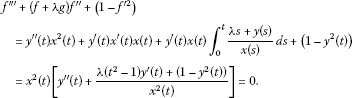This completes the proof. □

Remark 4.1 For $\lambda <-1$, by Theorem 1 , (1.1)-(1.3) has no solution such that ${lim}_{\eta \to \mathrm{\infty }}{g}^{\prime }\left(\eta \right)=1$ with $|{g}^{\prime }\left(\eta \right)|<1$ for $\eta \ge {\eta }_{0}$, ${\eta }_{0}\ge 0$ is a constant.

Utilizing the system (2.2)-(2.3), we know easily that (1.1)-(1.3) has no solution in Γ for $\lambda \le -1$.

In fact, if (1.1)-(1.3) has a solution $\left(f,g\right)\in \mathrm{\Gamma }$ for some $\lambda \le -1$, by Theorem 2.1, then (1.1)-(1.3) has a solution in $\left(x,y\right)\in Q$. Noticing that

we know

${g}^{″}\left(0\right)=x\left(0\right)={\int }_{0}^{1}\frac{\alpha \left(y\right)\left(s\right)\left(1-s\right)}{\phi x\left(s\right)}\phantom{\rule{0.2em}{0ex}}ds<0,$

This research uses integrals of equations to investigate the existence of solutions of the 3D axisymmetric inviscid stagnation flows related to Navier-Stokes equations and supplies a gap of analytical study in this field.

## References

1. Wang CY: Similarity stagnation point solutions of the Navier-Stokes equations-review and extension. Eur. J. Mech. B, Fluids 2008, 27: 678-683. 10.1016/j.euromechflu.2007.11.002

2. Davey A: A boundary layer flow at a saddle point of attachment. J. Fluid Mech. 1961, 10: 593-610. 10.1017/S0022112061000391

3. Howarth L: The boundary layer in three dimensional flow. Part II. The flow near a stagnation point. Philos. Mag. Ser. 7 1951, 42: 1433-1440.

4. Hiemenz K: Die Grenzschicht an einem in den gleichformingen Flussigkeitsstrom eingetauchten graden Kreiszylinder. Dinglers Polytech. J. 1911, 326: 321-324.

5. Homann F: Der Einfluss grosser Zahigkeit bei der Stromung um den Zylinder und um die Kugel. Z. Angew. Math. Mech. 1936, 16: 153-164. 10.1002/zamm.19360160304

6. Yang GC, Lan KQ: Nonexistence of the reversed flow solutions of the Falkner-Skan equations. Nonlinear Anal. TMA 2011, 74: 5327-5339. 10.1016/j.na.2011.05.017

7. Lan KQ, Yang GC: Positive solutions of a singular integral equation arising in boundary layer theory. Can. Math. Bull. 2008, 51(3):386-398. 10.4153/CMB-2008-039-7

8. Yang GC, Lan KQ: The velocity and shear stress functions of the Falkner-Skan equation arising in boundary layer theory. J. Math. Anal. Appl. 2007, 328: 1297-1308. 10.1016/j.jmaa.2006.06.042

9. Brighi B, Fruchard A, Sari T: On the Blasius problem. Adv. Differ. Equ. 2008, 13(5-6):509-600.

10. Padé O: On the solutions of the Falkner-Skan equations. J. Math. Anal. Appl. 2003, 285: 264-274. 10.1016/S0022-247X(03)00402-5

11. Wang CY: Review of similarity stretching exact solutions of the Navier-Stokes equations. Eur. J. Mech. B, Fluids 2011, 30: 475-479. 10.1016/j.euromechflu.2011.05.006

12. Deimling K: Nonlinear Functional Analysis. Spinger, New York; 1985.

## Acknowledgements

The authors wish to thank the anonymous referees for their valuable comments. This research was supported by the National Natural Science Foundation of China (Grant No. 11171046) and the Scientific Research Foundation of the Education Department of Sichuan Province, China.

## Author information

Authors

### Corresponding author

Correspondence to GC Yang.

### Competing interests

The authors declare that they have no competing interests.

### Authors’ contributions

All authors read and approved the final manuscript.

## Rights and permissions

Reprints and Permissions

Yang, G., Dang, L. & Xu, Y. Existence of solutions of a system of 3D axisymmetric inviscid stagnation flows. Bound Value Probl 2012, 153 (2012). https://doi.org/10.1186/1687-2770-2012-153

• Accepted:

• Published:

• DOI: https://doi.org/10.1186/1687-2770-2012-153

### Keywords

• Navier-Stokes equations
• 3D flows
• similarity solutions
• integral systems
• existence results